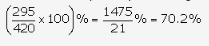# In a certain store, the profit is 320% of the cost. If the cost increases by 25%

In a certain store, the profit is 320% of the cost. If the cost increases by 25% but the selling price remains constant, approximately what percentage of the selling price is the profit?

Let CP =100.
Then, Profit =320, SP =420.
New CP = 125% of100 =125
New S.P. =420.
Profit =(420 - 125) =295
Therefore, required percentage =